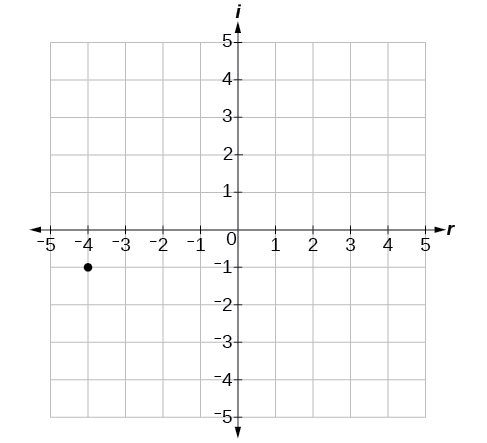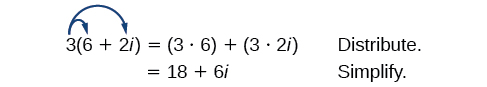# 2.4 Complex numbers  (Page 2/8)

 Page 2 / 8

Let’s consider the number $\text{\hspace{0.17em}}-2+3i.\text{\hspace{0.17em}}$ The real part of the complex number is $\text{\hspace{0.17em}}-2\text{\hspace{0.17em}}$ and the imaginary part is 3. We plot the ordered pair $\text{\hspace{0.17em}}\left(-2,3\right)\text{\hspace{0.17em}}$ to represent the complex number $\text{\hspace{0.17em}}-2+3i,$ as shown in [link] .

## Complex plane

In the complex plane, the horizontal axis is the real axis, and the vertical axis is the imaginary axis, as shown in [link] .

Given a complex number, represent its components on the complex plane.

1. Determine the real part and the imaginary part of the complex number.
2. Move along the horizontal axis to show the real part of the number.
3. Move parallel to the vertical axis to show the imaginary part of the number.
4. Plot the point.

## Plotting a complex number on the complex plane

Plot the complex number $\text{\hspace{0.17em}}3-4i\text{\hspace{0.17em}}$ on the complex plane.

The real part of the complex number is $\text{\hspace{0.17em}}3,$ and the imaginary part is –4. We plot the ordered pair $\text{\hspace{0.17em}}\left(3,-4\right)\text{\hspace{0.17em}}$ as shown in [link] .

Plot the complex number $\text{\hspace{0.17em}}-4-i\text{\hspace{0.17em}}$ on the complex plane.## Adding and subtracting complex numbers

Just as with real numbers, we can perform arithmetic operations on complex numbers. To add or subtract complex numbers, we combine the real parts and then combine the imaginary parts.

## Complex numbers: addition and subtraction

$\left(a+bi\right)+\left(c+di\right)=\left(a+c\right)+\left(b+d\right)i$

Subtracting complex numbers:

$\left(a+bi\right)-\left(c+di\right)=\left(a-c\right)+\left(b-d\right)i$

Given two complex numbers, find the sum or difference.

1. Identify the real and imaginary parts of each number.
2. Add or subtract the real parts.
3. Add or subtract the imaginary parts.

## Adding and subtracting complex numbers

1. $\left(3-4i\right)+\left(2+5i\right)$
2. $\left(-5+7i\right)-\left(-11+2i\right)$

1. $\begin{array}{ccc}\hfill \left(3-4i\right)+\left(2+5i\right)& =& 3-4i+2+5i\hfill \\ & =& 3+2+\left(-4i\right)+5i\hfill \\ & =& \left(3+2\right)+\left(-4+5\right)i\hfill \\ & =& 5+i\hfill \end{array}$

2. $\begin{array}{ccc}\hfill \left(-5+7i\right)-\left(-11+2i\right)& =& -5+7i+11-2i\hfill \\ & =& -5+11+7i-2i\hfill \\ & =& \left(-5+11\right)+\left(7-2\right)i\hfill \\ & =& 6+5i\hfill \end{array}$

Subtract $\text{\hspace{0.17em}}2+5i\text{\hspace{0.17em}}$ from $\text{\hspace{0.17em}}3–4i.$

$\left(3-4i\right)-\left(2+5i\right)=1-9i$

## Multiplying complex numbers

Multiplying complex numbers is much like multiplying binomials. The major difference is that we work with the real and imaginary parts separately.

## Multiplying a complex number by a real number

Lets begin by multiplying a complex number by a real number. We distribute the real number just as we would with a binomial. Consider, for example, $\text{\hspace{0.17em}}3\left(6+2i\right)$ :Given a complex number and a real number, multiply to find the product.

1. Use the distributive property.
2. Simplify.

## Multiplying a complex number by a real number

Find the product $\text{\hspace{0.17em}}4\left(2+5i\right).$

Distribute the 4.

$\begin{array}{ccc}\hfill 4\left(2+5i\right)& =& \left(4\cdot 2\right)+\left(4\cdot 5i\right)\hfill \\ & =& 8+20i\hfill \end{array}$

Find the product: $\text{\hspace{0.17em}}\frac{1}{2}\left(5-2i\right).$

$\frac{5}{2}-i$

## Multiplying complex numbers together

Now, let’s multiply two complex numbers. We can use either the distributive property or more specifically the FOIL method because we are dealing with binomials. Recall that FOIL is an acronym for multiplying First, Inner, Outer, and Last terms together. The difference with complex numbers is that when we get a squared term, $\text{\hspace{0.17em}}{i}^{2},$ it equals $\text{\hspace{0.17em}}-1.$

Given two complex numbers, multiply to find the product.

1. Use the distributive property or the FOIL method.
2. Remember that $\text{\hspace{0.17em}}{i}^{2}=-1.$
3. Group together the real terms and the imaginary terms

what are you up to?
nothing up todat yet
Miranda
hi
jai
hello
jai
Miranda Drice
jai
aap konsi country se ho
jai
which language is that
Miranda
I am living in india
jai
good
Miranda
what is the formula for calculating algebraic
I think the formula for calculating algebraic is the statement of the equality of two expression stimulate by a set of addition, multiplication, soustraction, division, raising to a power and extraction of Root. U believe by having those in the equation you will be in measure to calculate it
Miranda
state and prove Cayley hamilton therom
hello
Propessor
hi
Miranda
the Cayley hamilton Theorem state if A is a square matrix and if f(x) is its characterics polynomial then f(x)=0 in another ways evey square matrix is a root of its chatacteristics polynomial.
Miranda
hi
jai
hi Miranda
jai
thanks
Propessor
welcome
jai
What is algebra
algebra is a branch of the mathematics to calculate expressions follow.
Miranda
Miranda Drice would you mind teaching me mathematics? I think you are really good at math. I'm not good at it. In fact I hate it. 😅😅😅
Jeffrey
lolll who told you I'm good at it
Miranda
something seems to wispher me to my ear that u are good at it. lol
Jeffrey
lolllll if you say so
Miranda
but seriously, Im really bad at math. And I hate it. But you see, I downloaded this app two months ago hoping to master it.
Jeffrey
which grade are you in though
Miranda
oh woww I understand
Miranda
Jeffrey
Jeffrey
Miranda
how come you finished in college and you don't like math though
Miranda
gotta practice, holmie
Steve
if you never use it you won't be able to appreciate it
Steve
I don't know why. But Im trying to like it.
Jeffrey
yes steve. you're right
Jeffrey
so you better
Miranda
what is the solution of the given equation?
which equation
Miranda
I dont know. lol
Jeffrey
Miranda
Jeffrey
answer and questions in exercise 11.2 sums
how do u calculate inequality of irrational number?
Alaba
give me an example
Chris
and I will walk you through it
Chris
cos (-z)= cos z .
cos(- z)=cos z
Mustafa
what is a algebra
(x+x)3=?
6x
Obed
what is the identity of 1-cos²5x equal to?
__john __05
Kishu
Hi
Abdel
hi
Ye
hi
Nokwanda
C'est comment
Abdel
Hi
Amanda
hello
SORIE
Hiiii
Chinni
hello
Ranjay
hi
ANSHU
hiiii
Chinni
h r u friends
Chinni
yes
Hassan
so is their any Genius in mathematics here let chat guys and get to know each other's
SORIE
I speak French
Abdel
okay no problem since we gather here and get to know each other
SORIE
hi im stupid at math and just wanna join here
Yaona
lol nahhh none of us here are stupid it's just that we have Fast, Medium, and slow learner bro but we all going to work things out together
SORIE
it's 12
what is the function of sine with respect of cosine , graphically
tangent bruh
Steve
cosx.cos2x.cos4x.cos8x
sinx sin2x is linearly dependent
what is a reciprocal
The reciprocal of a number is 1 divided by a number. eg the reciprocal of 10 is 1/10 which is 0.1
Shemmy
Reciprocal is a pair of numbers that, when multiplied together, equal to 1. Example; the reciprocal of 3 is ⅓, because 3 multiplied by ⅓ is equal to 1
Jeza
each term in a sequence below is five times the previous term what is the eighth term in the sequence
I don't understand how radicals works pls
How look for the general solution of a trig functionByByBy OpenStaxBy Kimberly NicholsBy Anonymous UserBy Robert MurphyBy Dakota BocanBy Katy PrattBy Eric CrawfordByBy Richley CrapoBy Yacoub Jayoghli